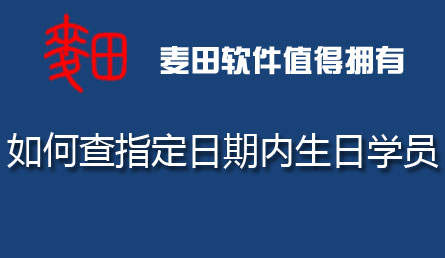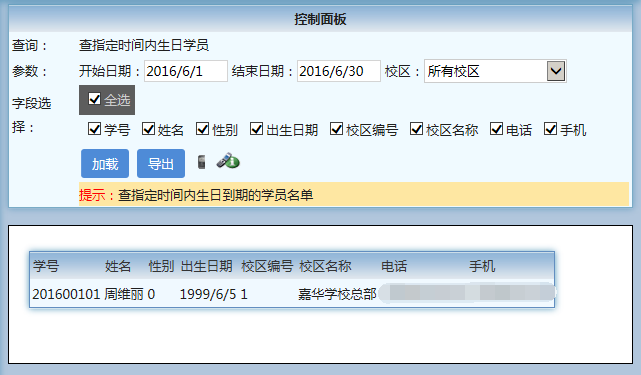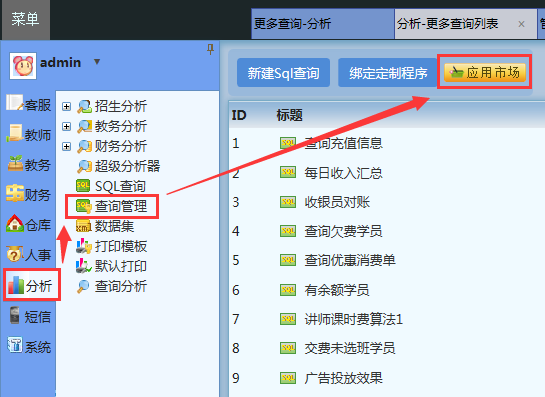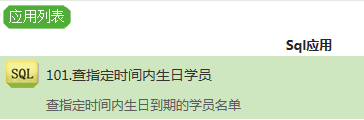查询篇-如何查指定日期内生日学员？-麦田软件

 2016年06月04日 02:21 点击率：4526【相关代码】

SQL版

Select
StudentID as 学号,
StudentName as 姓名,
Student.Sex as 性别,
Student.Birthday as 出生日期,
Student.SchoolID as 校区编号,
Student.SchoolName as 校区名称,
Student.Tel as 电话,
Student.MobileTel as 手机
from Student
where Birthday is not null
and Datepart(month, Birthday) >=Datepart(month, {@StartDate:开始日期})
and Datepart(month, Birthday) <= Datepart(month, {@EndDate:结束日期})
and Datepart(day, Birthday) >= Datepart(day, {@StartDate:开始日期})
and Datepart(day, Birthday) <= Datepart(day, {@EndDate:结束日期})
and Student.SchoolID in ( {@:校区})

Acess版

Select
StudentID as 学号,
StudentName as 姓名,
Student.Sex as 性别,
Student.Birthday as 出生日期,
Student.SchoolID as 校区编号,
Student.SchoolName as 校区名称,
Student.Tel as 电话,
Student.MobileTel as 手机
from Student
where Birthday is not null
and Datepart('d', Birthday) >=Datepart('d', {@StartDate:开始日期})
and Datepart('d', Birthday) <= Datepart('d', {@EndDate:结束日期})
and Datepart('M', Birthday) >= Datepart('M', {@StartDate:开始日期})
and Datepart('M', Birthday) <= Datepart('M', {@EndDate:结束日期})
and Student.SchoolID in ( {@SchoolID:校区})【关于麦田】